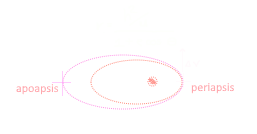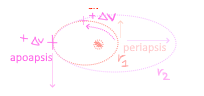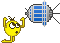# Equation for Periapsis Height During Apoapsis Burn???

#### 5kinflute

##### New member
What is the equation for increasing Periapsis Height during Apoapsis Burn ???

Hi, I am stuck at a problem I can't figure out at all, and it has to do with a space-craft conducting a burn once it is at APOAPSIS.figure 1
With the equation above, where the angle to the spacecraft is Θ = 0° ; when I increase my current velocity from 1660 km/s to 2000 km/s ... my Apoasis increases and the formula checks out.

The equation is a problem because what if I am at Apoapsis and want to increase my Periapsis altitude using an equation, just like the image below:figure 2

I have reviewed websites such as this link: http://www.braeunig.us/space/orbmech.htm#elements under motions of planets and satellites
but this is a problem when I program this using the equation above... Vp, Va, Rp, Ra and e are all dependent on each other ... with the following
relationship:

$r_{p}v_{p}=r_{a}v_{a}$ where e = [imath]\frac{r_{p}v^{2}_{p}}{GM}-1[/imath]

given 3D vectors [imath]\acute{v}[/imath] and [imath]\acute{r}[/imath] of a spacecraft, when I move these values such that it is at apopasis... the apopasis
continues to grow when increasing delta V to conduct hohmann's transfer

PLEASE HELP...•N_Molson

#### martins

##### Orbiter Founder
Orbiter Founder
You probably want to look at the vis-viva equation here:
$v = \sqrt{\mu \left(\frac{2}{r} - \frac{1}{a} \right) }$From this you can calculate the orbital speed at apoapsis of your current orbit ([imath]v_{ap} = v(r_{ap})[/imath])
Likewise, you can calculate the orbital speed at periapsis of the target orbit (assuming [imath]r_{ap}(current) = r_{pe}(target)[/imath])
The required delta-v for the burn is then simply the difference between the two.

••kuddel, Urwumpe, francisdrake and 1 other person

#### MontBlanc2012

##### Member
Here are a couple of useful equations:

\begin{aligned} v_a &= \sqrt{\frac{r_p}{r_a}\,\frac{2\,\mu}{r_a + r_p}} \\ v_p &= \sqrt{\frac{r_a}{r_p}\,\frac{2\,\mu}{r_a + r_p}} \\ \end{aligned}
Now, let’s suppose that one executes a prograde burn at apoapsis of magnitude [imath]\delta V[/imath]; and let’s suppose that the initial periapsis radius is [imath]r_{p,i}[/imath] and is raised to a final periapsis radius, [imath]r_{p,f}[/imath] at the end of the burn. It follows from the above equations that:

\begin{aligned} v_a &= \sqrt{\frac{r_{p,i}}{r_a}\,\frac{2\,\mu}{r_a + r_{p,i}}} \\ v_a + \delta V &= \sqrt{\frac{r_{p,f}}{r_a}\,\frac{2\,\mu}{r_a + r_{p,f}}} \\ \end{aligned}
We can eliminate [imath]v_a[/imath] from these equations and solve for [imath]\delta V[/imath] to give:

$\delta V = \sqrt{\frac{2\,\mu}{r_a}}\,\left(\sqrt{\frac{r_{p,f}}{r_a + r_{p,f}}} -\sqrt{\frac{r_{p,i}}{r_a + r_{p,i}}}\right)$
In other words, this is the expression for the dV required to raise the periapsis from [imath]r_{p,i}[/imath] to [imath]r_{p,f}[/imath] for a prograde burn executed at apoapsis.

Last edited:
••kuddel, francisdrake and N_Molson

thank you!!!

#### EstebanDF

##### Member
Here are a couple of useful equations:
...
We can eliminate [imath]v_a[/imath] from these equations and solve for [imath]\delta V[/imath] to give:

$\delta V = \sqrt{\frac{2\,\mu}{r_a}}\,\left(\sqrt{\frac{r_{p,f}}{r_a + r_{p,f}}} -\sqrt{\frac{r_{p,i}}{r_a + r_{p,i}}}\right)$
In other words, this is the expression for the dV required to raise the periapsis from [imath]r_{p,i}[/imath] to [imath]r_{p,f}[/imath] for a prograde burn executed at apoapsis.
Awesome, tested the formula with the BurnTimeMFD v3.1 and got 400 mt. short (Calculated Dv was 28.49055788, so the 0.00055788 is the reason)